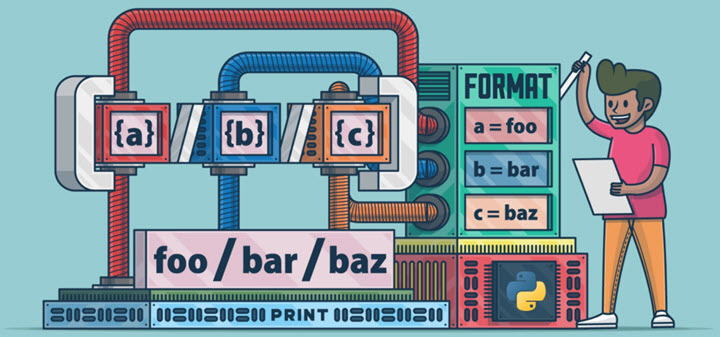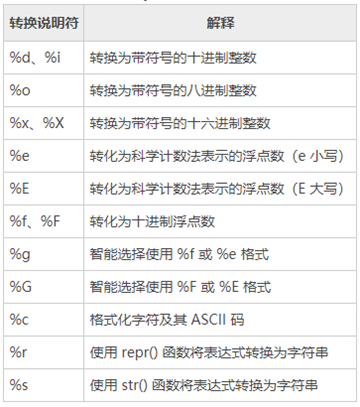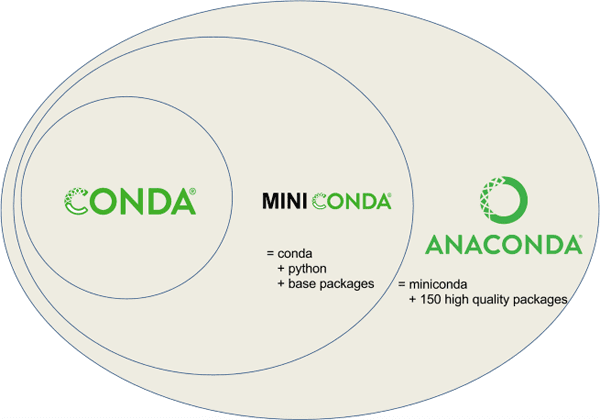# Python字符串格式化工具## %格式化工具• %o —— oct 八进制
• %d —— dec 十进制
• %x —— hex 十六进制

• %f ——保留小数点后面六位有效数字。%.3f，保留3位小数位
• %e ——保留小数点后面六位有效数字，指数形式输出。%.3e，保留3位小数位，使用科学计数法
• %g ——在保证六位有效数字的前提下，使用小数方式，否则使用科学计数法。%.3g，保留3位有效数字，使用小数或科学计数法

• %s ——输出字符串
• %10s ——右对齐，占位符10位
• %-10s ——左对齐，占位符10位
• %.2s ——截取2位字符串
• %10.2s ——10位占位符，截取两位字符串
print('%o' % 20)
print('%d' % 20)
print('%x' % 20)

print('%f' % 1.11)  # 默认保留6位小数
print('%.1f' % 1.11)  # 取1位小数
print('%e' % 1.11)  # 默认6位小数，用科学计数法
print('%.3e' % 1.11)  # 取3位小数，用科学计数法
print('%g' % 1111.1111)  # 默认6位有效数字
print('%.7g' % 1111.1111)  # 取7位有效数字
print('%.2g' % 1111.1111)  # 取2位有效数字，自动转换为科学计数法

print('%s' % 'hello world')  # 字符串输出
print('%20s' % 'hello world')  # 右对齐，取20位，不够则补位
print('%-20s' % 'hello world')  # 左对齐，取20位，不够则补位
print('%.2s' % 'hello world')  # 取2位
print('%10.2s' % 'hello world')  # 右对齐，取2位
print('%-10.2s' % 'hello world')  # 左对齐，取2位

# 输出：
# 24
# 20
# 14
# 1.110000
# 1.1
# 1.110000e+00
# 1.110e+00
# 1111.11
# 1111.111
# 1.1e+03
# hello world
#          hello world
# hello world
# he
#         he
# he


## format 函数

str.format()增强了字符串格式化的功能。基本语法是通过 {} 和 : 来代替以前的 % 。format 函数可以接受不限个参数，位置可以不按顺序。

• 不带编号，即“{}”
• 带数字编号，可调换顺序，即“{1}”、“{2}”
• 带关键字，即“{a}”、“{tom}”

• ‘b’ – 二进制。将数字以2为基数进行输出。
• ‘c’ – 字符。在打印之前将整数转换成对应的Unicode字符串。
• ‘d’ – 十进制整数。将数字以10为基数进行输出。
• ‘o’ – 八进制。将数字以8为基数进行输出。
• ‘x’ – 十六进制。将数字以16为基数进行输出，9以上的位数用小写字母。
• ‘e’ – 幂符号。用科学计数法打印数字。用’e’表示幂。
• ‘g’ – 一般格式。将数值以fixed-point格式输出。当数值特别大的时候，用幂形式打印。
• ‘n’ – 数字。当值为整数时和’d’相同，值为浮点数时和’g’相同。不同的是它会根据区域设置插入数字分隔符。
• ‘%’ – 百分数。将数值乘以100然后以fixed-point(‘f’)格式打印，值后面会有一个百分号。

• < （默认）左对齐、> 右对齐、^ 中间对齐、= （只用于数字）在小数点后进行补齐
• 取位数“{:4s}”、”{:.2f}”等

import datetime

print('{} {}'.format('hello', 'world'))  # 不带字段
print('{0} {1}'.format('hello', 'world'))  # 带数字编号
print('{0} {1} {0}'.format('hello', 'world'))  # 打乱顺序
print('{1} {1} {0}'.format('hello', 'world'))
print('{a} {tom} {a}'.format(tom='hello', a='world'))  # 带关键字

print('{0:b}'.format(3))
print('{:c}'.format(20))
print('{:d}'.format(20))
print('{:o}'.format(20))
print('{:x}'.format(20))
print('{:e}'.format(20))
print('{:g}'.format(20.1))
print('{:f}'.format(20))
print('{:n}'.format(20))
print('{:%}'.format(20))

print('{} and {}'.format('hello', 'world'))  # 默认左对齐
print('{:10s} and {:>10s}'.format('hello', 'world'))  # 取10位左对齐，取10位右对齐
print('{:^10s} and {:^10s}'.format('hello', 'world'))  # 取10位中间对齐
print('{} is {:.2f}'.format(1.123, 1.123))  # 取2位小数
print('{0} is {0:>10.2f}'.format(1.123))  # 取2位小数，右对齐，取10位
print('{:<30}'.format('left aligned'))  # 左对齐
print('{:>30}'.format('right aligned'))  # 右对齐
print('{:^30}'.format('centered'))  # 中间对齐
print('{:*^30}'.format('centered'))  # 使用“*”填充
print('{:0=30}'.format(11))  # 还有“=”只能应用于数字，这种方法可用“>”代替

print('{:+f}; {:+f}'.format(3.14, -3.14))  # 总是显示符号
print('{: f}; {: f}'.format(3.14, -3.14))  # 若是+数，则在前面留空格
print('{:-f}; {:-f}'.format(3.14, -3.14))  # -数时显示-，与'{:f}; {:f}'一致

print('{:,}'.format(1234567890))  # 数字千位分隔符

print('{:%Y-%m-%d %H:%M:%S}'.format(datetime.date.today()))  # 日期时间格式化


## 字符串插值F-strings

# Python程序演示
# 字符串插值
n1 = 'Hello'
n2 ='GeeksforGeeks'
# f告诉Python恢复大括号{}中两个字符串变量名和程序的值
print(f"{n1}! This is {n2}")
# 内联算法
print(f"(2 * 3)-10 = {(2 * 3)-10}")


## 拓展：字符串模板

string.Template，将一个string设置为模板，通过替换变量的方法，最终得到想要的string。

from string import Template

template_string = '$who likes$what'
s = Template(template_string)
d = {'who': 'Tim', 'what': 'Kong Fu'}
s_out = s.substitute(d)
print(s_out)##### 使用Excel搭建推荐系统##### Python数据科学IDE软件的选择